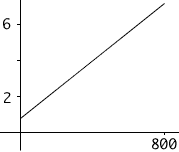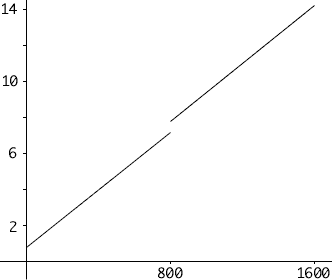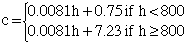Subject: Linear Equation Name: Jacky Lam Who is asking: Student Level: Secondary Question: I encountered this question in my text book. There is a light bulb and it is given that the light bulb cost \$0.75 and the cost of operating it is \$0.0081 per hour. From the information give, I came up with the linear equation: Let c be total cost and Let h be hours used. Therefore: c = 0.0081h + 0.75 represents the total cost of the light bulb and the electricity. However, the second part of the question added the fact that the light bulb will only last for 800 hours. If the light bulb is replaced as soon as it burns out exactly after every 800 hours, how can I write an equation that represents that? Is it possible? What would it look like on the graph. Thanks for your kind attention. Jacky Hi Jacky, In the first 800 hours the function c = 0.0081h + 0.75 has graphand at h = 800 hours the tatal cost is \$6.48 For part 2 of the question, at 800 hours there is an immediate cost of \$0.75 for a new light bulb increasing the total cost to \$6.48 + \$0.75 = \$7.23 and then the cost of \$0.0081 per hour continues. Hence, geometrically what you have isTo write this algebrically it is easiest to break the algebra into two expressions just as the graph naturally falls into two pieces. For the first 800 hours the cost is given by c = 0.0081h + 0.75 and for the next 800 hours the cost is given by c = 0.0081h + 7.23 This can be writtenHarley Go to Math Central